Shubham Kumar ShuklaShubham9455

15min

# TIME SERIES FORECASTING using Python

There are many questions that come to mind with a topic like time series forecasting. Questions like: What is time series forecasting? Why do we use time series forecasting? Where do we use time series forecasting? When will we not use time series forecasting? In this article you are going to learn the answers to these questions.

### What is time series forecasting?

Time series forecasting is like other machine learning algorithms used for prediction of any dependent values or features. In supervised machine learning there are many algorithms like linear and logistic regression which we use to predict output with the help of mapping functions, which map dependent to independent values. But, in time series forecasting we have only one variable that is time corresponding, to which we train our model and predict the future values with the help of previous time data. We do this because there is some type of pattern or trend found in our time data in measured intervals. We use this to analyze our time data.

Time series is a set of observations taken at specified times, usually at equal intervals like day, month, week, year, or any measure of time. It is used to predict future values on the basis of previous observed values.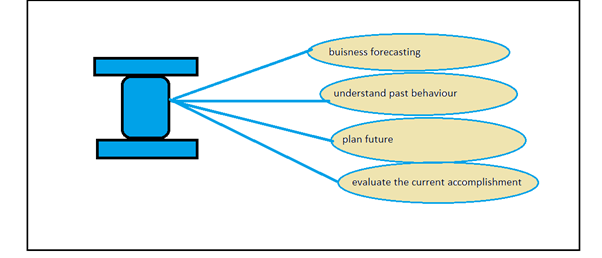From the above we see that we can use time series forecasting for things like business models, weather forecasting, sales forecasting,or pandemic forecasting over an instance of time.

1. Business forecasting

2. Weather forecasting

3. Pandemic forecasting

There are many components in time series forecasting like trends, seasonality, irregularity, and cyclic.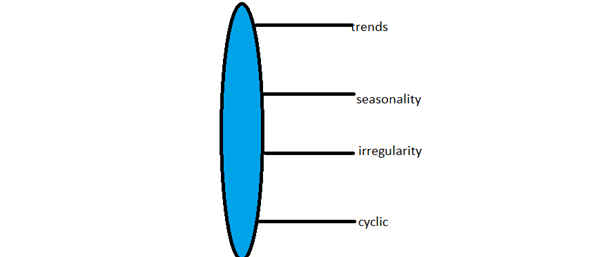Trend: Trend is a movement to relative higher or lower values over a long period of time.

Trends can be upward or downward and are an overall look at our data. A trend is always a relative view of data.

Seasonality: Seasonality is an upward or downward swing for a period of time. For example, every December for the Christmas season the sale of decorative objects and chocolates increases, so every December is the seasonality of our dataset.

Irregularity: Irregularities are short-duration or non-repeating events, like the Covid-19 pandemic. This disturbed the whole pattern of our environment so the pandemic duration is known as an irregularity in our dataset. This can happen any time.

Cyclic: Cyclic means data moving upward or downward and repeating that pattern after some time.

#### When to not to use time series forecasting

There are many situations where we do not use time series forecasting, like when the values are constant.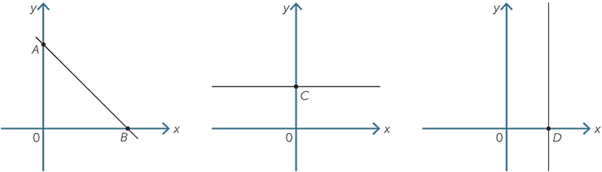When the values are in the form of a function like sin(x) or cos(x)

#### Stationarity

Stationarity means the model generates some output with respect to some interval of time. It remains constant or does not change with respect to the time. Here it does not mean the values of the graph remain constant, it means the graph follows the same pattern of seasonality over a particular instance of time. The below illustrates the concept of seasonality.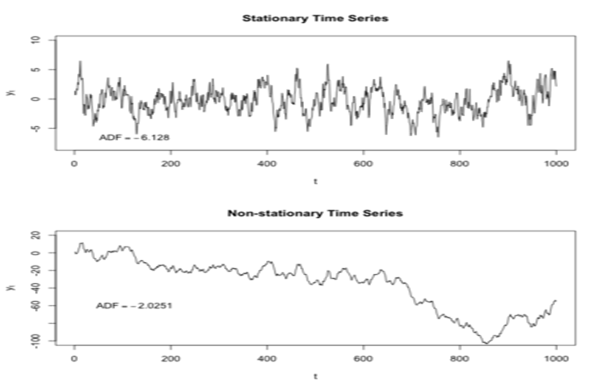What is stationarity?

Time series show a particular behavior over time with high probability that it will follow the same in the future. It is important to remove seasonality. If we do not remove it, it will create problems in future prediction.

There are many ways to detect seasonality.

1. Constant mean: There is a constant mean of data over some interval of time.

2. Constant variance : The variance of the data is constant over some specific interval of time.

Variance is a second order differentiation.

1. Auto-variance: Auto-variance does not depend on time when the values do not have to depend on each other.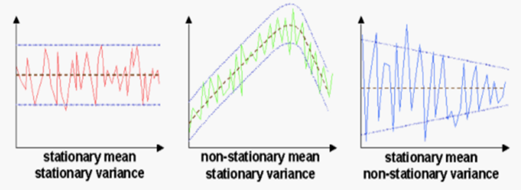There is a lot of information on stationarity, like trends stationarity, seasonality stationarity, moving average stationarity, and auto-regressive stationarity. But keep one point clear in your mind: Stationarity means over some particular instance of time when the value of data does not change. Here values of data are relative, which means there is the same pattern in the graph over a particular instance of time. I will create a separate article on this topic to help you understand stationarity better.

Methods to check stationarity.

1. Rolling statistics: Draw the moving average or moving variance to visualize if values change with a duration of time. Rolling statistics data is divided into various parts of statistical properties like mean, median, mode or variance, which are moving or changing with respect to time.

2. Moving average

3. Trailing average

4. Auto-regressive

5. Auto-regressive moving model

Now below we see all the above components of rolling statistics.

1. Moving average:

In moving average we simply take the average of our values with respect to a sliding window.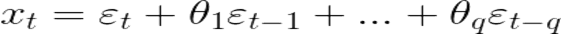From the above we see that the function is linear and et is noise function we will insert into our model for checking the stationarity.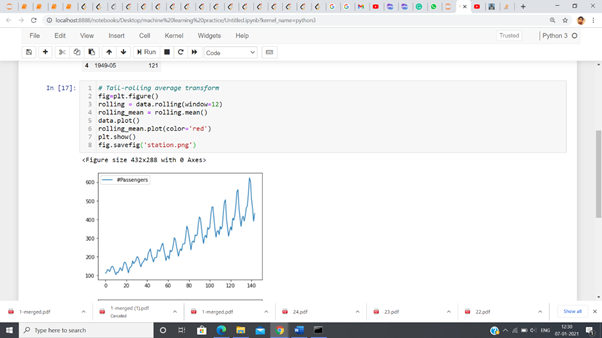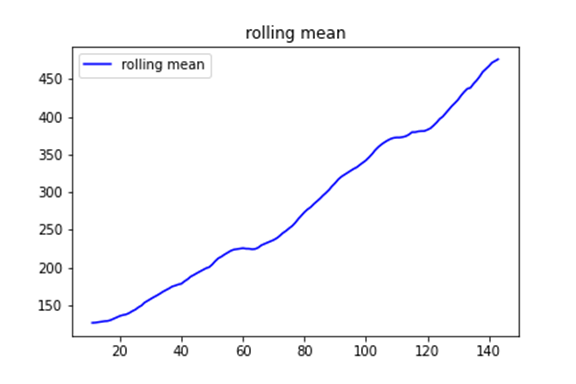1. Auto-regressive: Auto-regressive uses the past value for prediction and adds the random noise/error into it.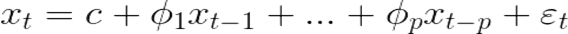Here Φ is weight and et is noise in our data––

Code implementation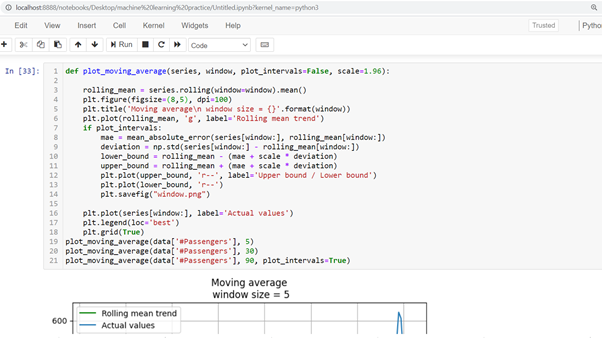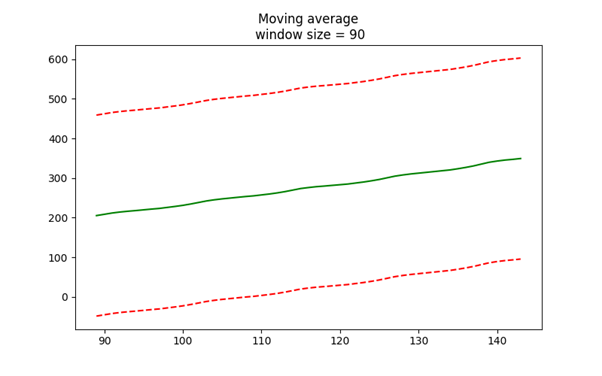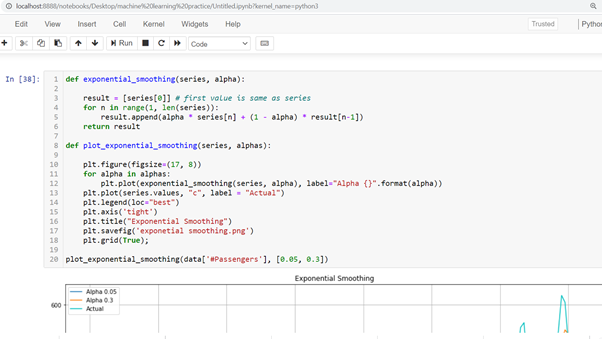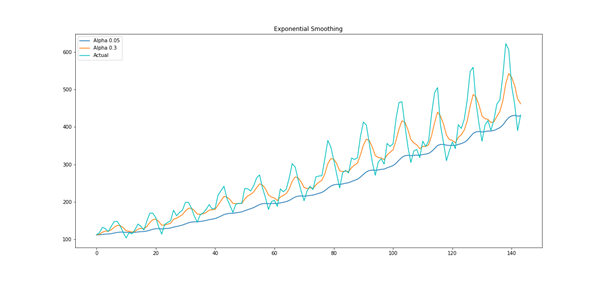Here we see different window sizes of how many parts of data we want to take to calculate moving averages on that part.

1. Auto-regressive moving average: This is the combination of both moving average and auto-regression. This is also called the ARMA model which in the past was part of the ARIMA model.

ARMA(p, q)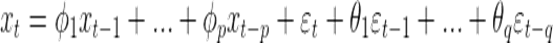Below you will see how our non-seasonality looks after applying different models.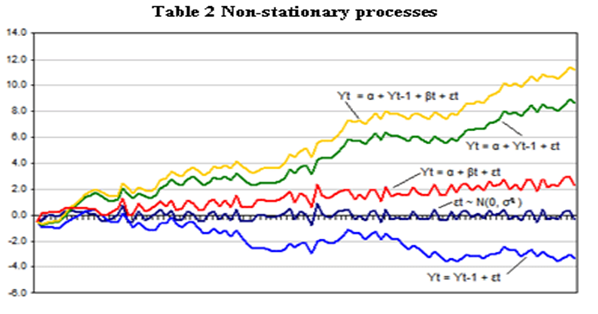When we apply calculus in our model this converts into ARIMA model.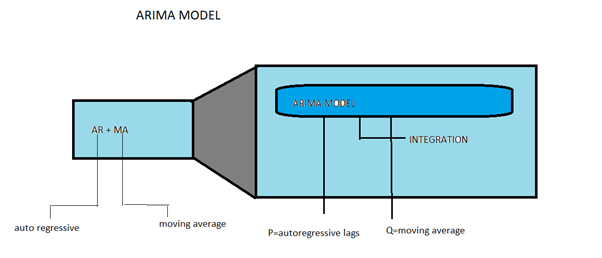Next we will see everything that is here in code implemented view

2. ADCF: The next step for checking seasonality is the augmented dickey-fuller test. This library of stats-models, which is a predefined library for time series forecasting, is used to measure the nonlinear dependency on time data. This consists of test-statistics and some critical values.

yt=α+βt+ϕyt−1+et

Below is the code implementation of the ACDF test.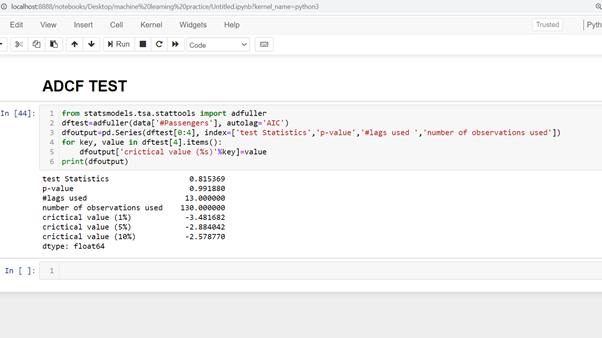I will conclude that time series forecasting is a very large and interesting topic and the whole thing is based on mathematics. There are many good textbooks on the subject, but one of the best ones is mentioned below.

References:

A lot of the content in this article was written by me but there are some references mentioned below:

Introduction to time series forecasting

The Complete Guide to Time Series Analysis and Forecasting

https://towardsdatascience.com/the-complete-guide-to-time-series-analysis-and-forecasting-70d476bfe775

An Introductory Study on Time Series Modeling and Forecasting

https://arxiv.org/ftp/arxiv/papers/1302/1302.6613.pdf